# Quick math

Do not overlook the mathematical tricks behind the seemingly simple equations in this game - you may need to spend more time than you think to complete them! In Quick Math, your goal is to fill in the missing arithmetic symbols in the equations as quickly as you can. You will be presented a simple equation at each round, with the four arithmetic symbols of addition, subtraction, multiplication and division shown underneath. Click the correct symbol to fill the blank in the equation and make it valid. The time you have spent for each question is recorded at the top of the screen, and your score will be counted based on it. If you have clicked a wrong symbol, 1000 points will be deducted, but you may still complete all 20 questions in the game. Come and challenge your reaction speed and judgment, but try not to hesitate or second-guess yourself!

## Related software (5)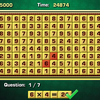## Arithmetic Game

Complete the equations in the interesting arithmetic test!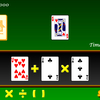## Make 24

Use the given cards to form a valid equation within 60 seconds!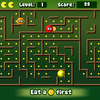## Math Man

Play Ghost Man with a mathematical twist!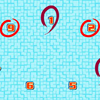## Make 15

Pick 3 numbers that add up to 15.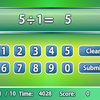## Quick Calculate

Showcase your arithmetic skills to get the answers!